### Home > CAAC > Chapter 14 > Lesson 14.2.1.4 > Problem2-55

2-55.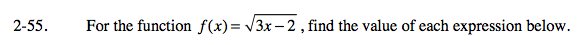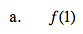Substitute 1 for x.

$f(1)=\sqrt{3-2}$

f(1) = 1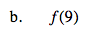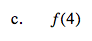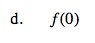The square root of a negative number is undefined.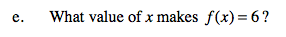Substitute 6 for f(x).

$6 = \sqrt{3\textit{x}-2}$

Square both sides.

36 = 3x − 2

Solve for x.

$\textit{x}=12\frac{2}{3}$